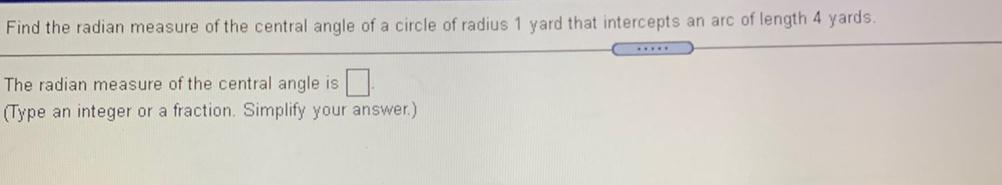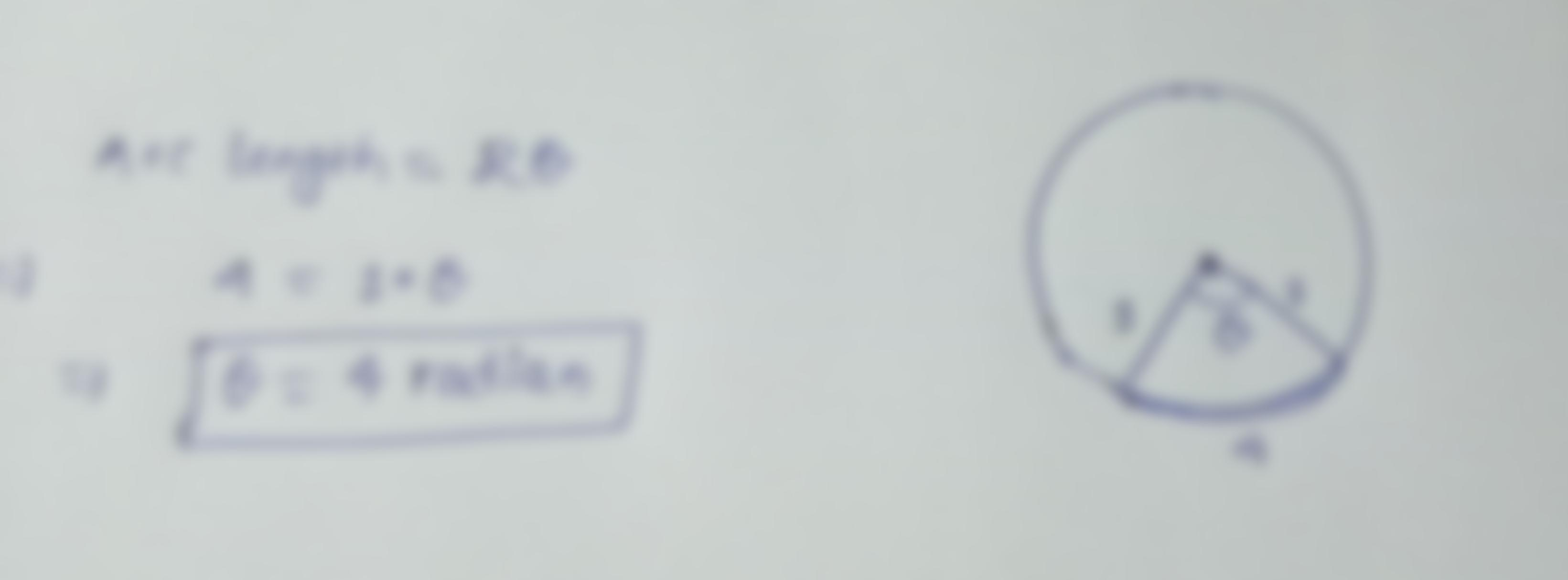Question:

Find the radian measure of the central angle of a circle of radius 1 yard that intercepts an arc of length 4 yards. The radian mFind the radian measure of the central angle of a circle of radius 1 yard that intercepts an arc of length 4 yards. The radian measure of the central angle is I (Type an integer or a fraction. Simplify your answer.)